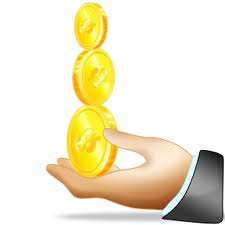### 3. In each of the following experiments, write the sample space S, number of sample point n (S), events A, B and n(A), n(B). A coin is tossed three times. A is the event that head appears once, B is the event that head appears at the most twice.

 Since a coin is tossed three times∴ Sample space = {HHH, HHT, HTH, HTT, THH, THT, TTH, TTT} ∴ n(S) = 8 Let A = Event that the head appears once. ∴ A = {HTT, THT, TTH } ∴ n(A) = 3 Let B = Event that the head appears at the most twice ∴ B = {HHT, HTH, HTT, THH, THT, TTH, TTT} ∴ n(B) = 7